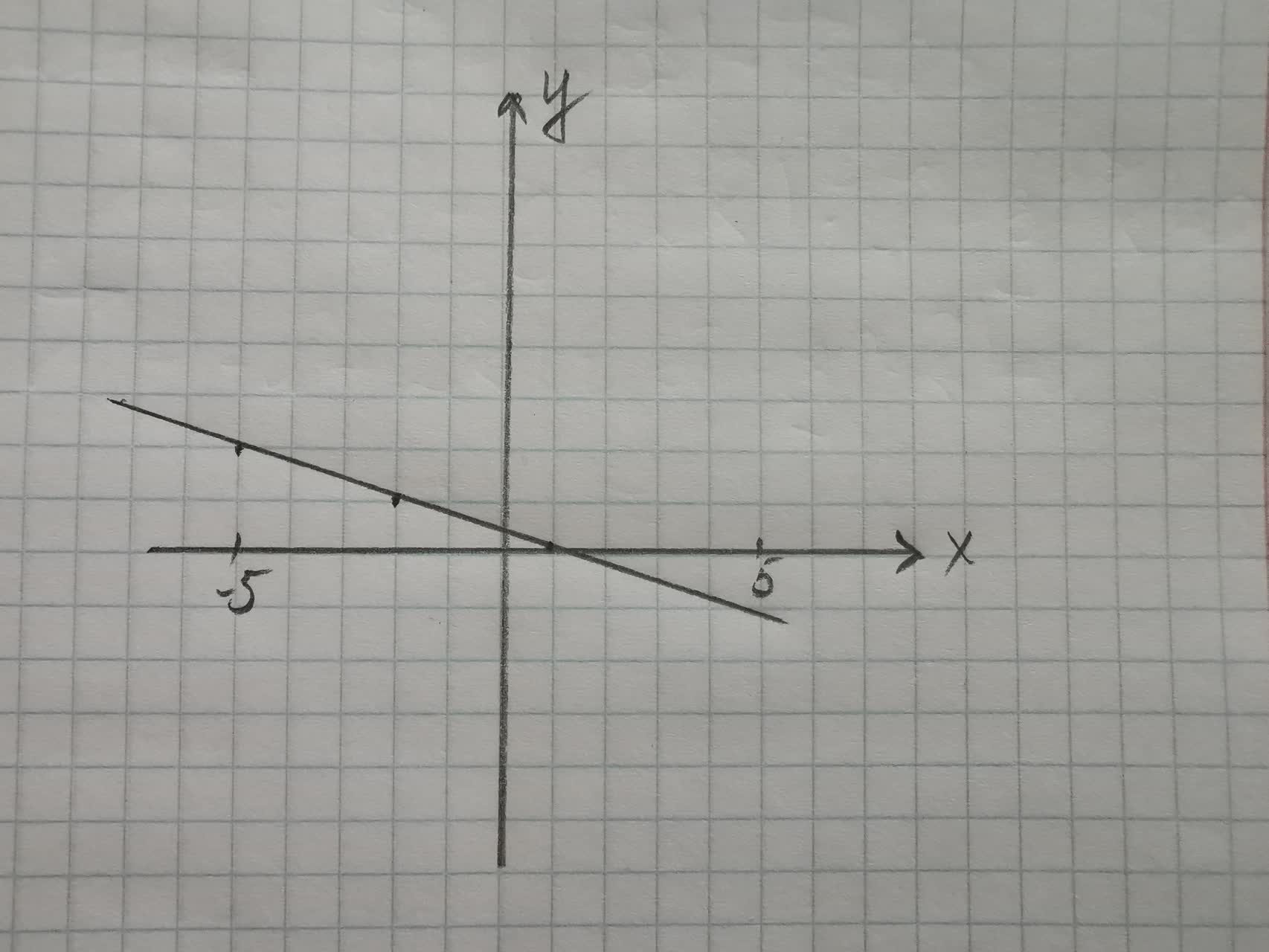Recent questions in Parametric equations, polar coordinates, and vector-v

Recent questions in Parametric equations, polar coordinates, and vector-vTyra 2021-09-06 Answered

Find a parametric vector equation for the given plane:Clifland 2021-09-05 Answered

Using the point $$\displaystyle{P}_{{{0}}}={\left({1},{0},-{2}\right)}$$ and the vector $$v=(1,3,-1)$$ write a parametric vector equation for a line through $$\displaystyle{P}_{{{0}}}$$ in the direction of vYasmin 2021-08-30 Answered

Write the scalar equation for a plane through the point $$\displaystyle{P}_{{{0}}}={\left({1},{0},-{2}\right)}$$ with normal vector $$v=(1,3,-1)$$Reeves 2021-08-29 Answered

$$x=t-\sin t$$ $$y=1-\cos t$$ to the curve given by the parametric equation $$\displaystyle{t}={\frac{{\pi}}{{{3}}}}$$ find teh equation of the line that is tangent at its pointusagirl007A 2021-08-22 Answered

Consider the curve in the plane given in polar coordinates by $$\displaystyle{r}={4}{\sin{\theta}}$$. Find the cartesian equation for the curve and identify the curvehexacordoK 2021-08-22 Answered

The position of an object in circular motion is modeled by the parametric equations $$\displaystyle{x}={3}{\sin{{2}}}{t}$$ $$\displaystyle{y}={3}{\cos{{2}}}{t}$$ where t is measured in seconds. Describe the path of the object by stating the radius of the circle, the position at time t = 0, the orientation of motion (clockwise or counterclockwise), and the time t it takes to complete one revolution around the circle.Maiclubk 2021-08-22 Answered

Parametric equations and a value for the parameter t are given $$\displaystyle{x}={\left({80}{\cos{{45}}}^{{o}}\right)}{t},{y}={6}+{\left({80}{\sin{{45}}}^{{o}}\right)}{t}-{16}{t}^{{2}}$$. t = 2. Find the coordinates of the point on the plane curve described by the parametric equations corresponding to the given value of t.shadsiei 2021-08-22 Answered

Parametric equations and a value for the parameter t are given x = 3 - 5t, y = 4 + 2t. t = 1. Find the coordinates of the point on the plane curve described by the parametric equations corresponding to the given value of t.Reggie 2021-08-21 Answered

Graph the sets of points whose polar coordinates satisfy the equations and inequalities $$\displaystyle{1}\le{r}\le{2}$$preprekomW 2021-08-21 Answered

Given $$\displaystyle{u}=<{3},{5}>{\quad\text{and}\quad}{v}=<{6},{10}>$$, find the magnitude of the vector and find the dot product.Brennan Flores 2021-08-20 Answered

Graph the sets of points whose polar coordinates satisfy the equations and inequalities r = 2slaggingV 2021-08-20 Answered

Give two pairs of parametric equations that generate a circle centered at the origin with radius 6.BenoguigoliB 2021-08-20 Answered

Parametric equations and a value for the parameter t are given $$\displaystyle{x}={t}^{{2}}+{3},{y}={6}-{t}^{{3}}$$.t=2 Find the coordinates of the point on the plane curve described by the parametric equations corresponding to the given value of t.Tolnaio 2021-08-19 Answered

Graph the sets pf points whose polar coordinates satisfy the equations and inequalities $$\displaystyle\theta=\frac{\pi}{{2}},{r}\ge{0}$$e1s2kat26 2021-08-19 Answered

A curve is given by the following parametric equations. $$\displaystyle{x}={20}{\cos{{t}}},{y}={10}{\sin{{t}}}$$. The parametric equations are used to represent the location of a car going around the racetrack. a) What is the cartesian equation that represents the race track the car is traveling on? b) What parametric equations would we use to make the car go 3 times faster on the same track? c) What parametric equations would we use to make the car go half as fast on the same track?nagasenaz 2021-08-18 Answered

Change from rectangular to cylindrical coordinates. (Let $$\displaystyle{r}\geq{0}$$ and $$\displaystyle{0}\leq\theta\leq{2}\pi$$.) a) $$\displaystyle{\left(-{2},{2},{2}\right)}$$ b) $$\displaystyle{\left(-{9},{9}\sqrt{{{3},{6}}}\right)}$$ c) Use cylindrical coordinates. Evaluate $$\displaystyle\int\int\int_{{{E}}}{x}{d}{V}$$ where E is enclosed by the planes $$\displaystyle{z}={0}$$ and $$\displaystyle{z}={x}+{y}+{10}$$ and by the cylinders $$\displaystyle{x}^{{{2}}}+{y}^{{{2}}}={16}$$ and $$\displaystyle{x}^{{{2}}}+{y}^{{{2}}}={36}$$ d) Use cylindrical coordinates. Find the volume of the solid that is enclosed by the cone $$\displaystyle{z}=\sqrt{{{x}^{{{2}}}+{y}^{{{2}}}}}$$ and the sphere $$\displaystyle{x}^{{{2}}}+{y}^{{{2}}}+{z}^{{{2}}}={8}$$.aflacatn 2021-08-16 Answered

Is it true that the equations r=8, $$\displaystyle{x}^{{2}}+{y}^{{2}}={64}$$, and $$\displaystyle{x}={8}{\sin{{\left({3}{t}\right)}}},{y}={8}{\cos{{\left({3}{t}\right)}}}{\left({0}\le{t}\le{2}\pi\right)}$$ all have the same graph.Albarellak 2021-08-16 Answered

Let C be the curve described by the parametric equations x(t)= t, y(t) =$$\displaystyle{t}^{{3}}$$ a) sketch a graph of C b) find the vector-valued function f(t) associated with this parameterization of the curve C.amanf 2021-08-15 Answered

Graph the sets of points whose polar coordinates satisfy the equations and inequalities $$\displaystyle{r}\ge{1}$$Wotzdorfg 2021-08-11 Answered

...